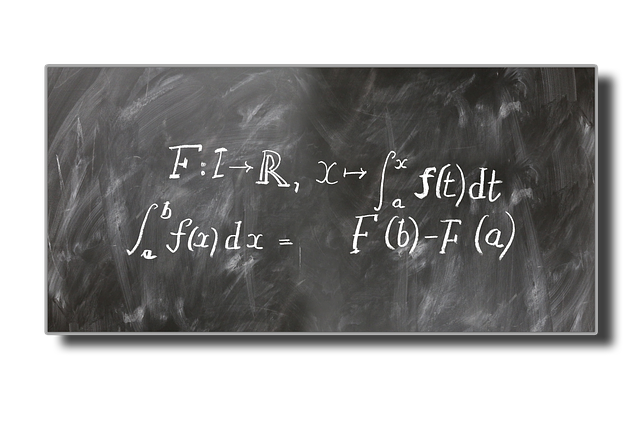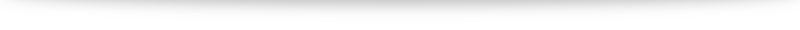# Integration techniques

1. Equations and inequalities
2. Curves and transformations
3. Functions
4. Arithmetic and geometric progressions
5. Series and the sigma notation
6. Differentiation
7. Maclaurin series and binomial expansion
8. Integration techniques
9. Definite integrals
10. Differential equations
11. Vectors
12. Complex numbers

Next up: randomly generated question on integration to test your concepts. Then vectors, which will likely take a whole chunk of time and effort. Let’s work hard together!

Picture credit: Pixabay and geralt .
Some further discussion for those interested:
When we write $\int f(x) \; \mathrm{d}x$, we call the result the (indefinite) integral of $f(x)$. At higher levels, we typically denote our result by the expression “$F(x)$” and call it the “anti-derivative” of $f(x)$. Integration, on the other hand, refers to a process of taking the limits of a special type of sum (at least in the context of Riemann integrals). We will see a glimpse of this in chapter 9. A beautiful result, called the “fundamental theorem of calculus”, says that the processes of differentiation and integration are opposites (with a few technical qualifiers). In other words, integration is no different from anti-differentiation: the naming convention we adopt is still correct (albeit with quite a bit swept under the carpet).